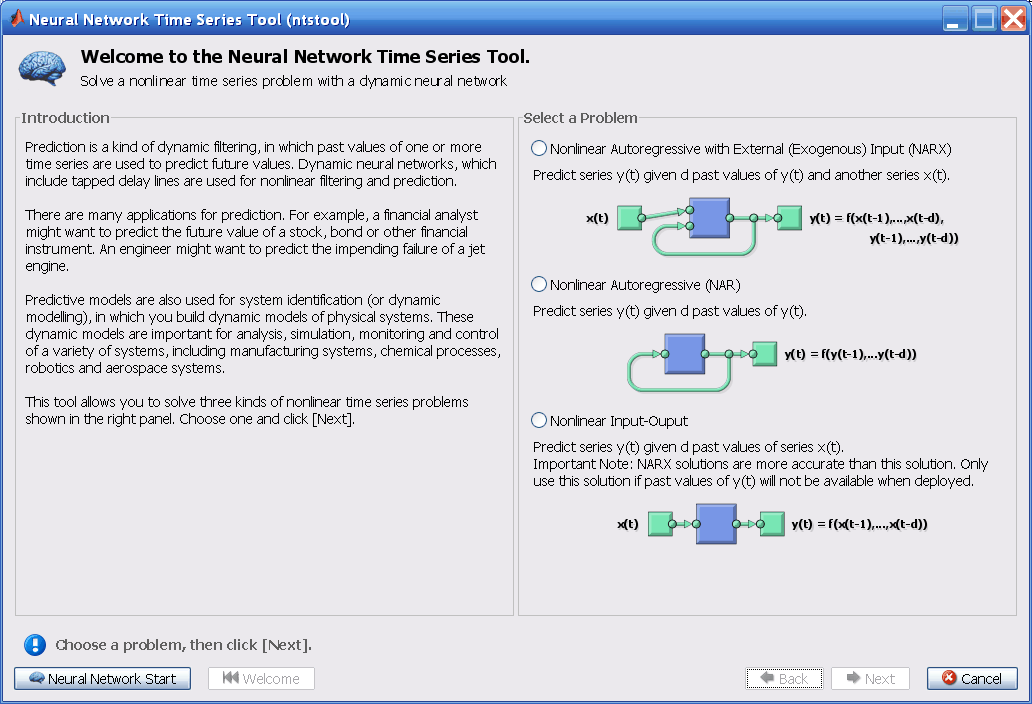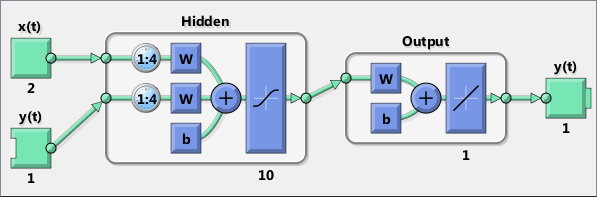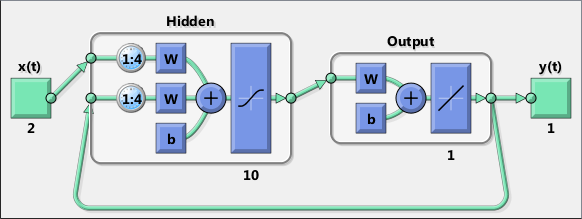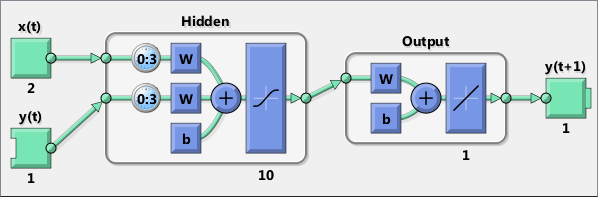@devilogic 2016-08-13T09:17:51.000000Z 字数 4138 阅读 2931

# 通过神经网络进行时序预测与建模（基础应用四）

matlab

## 定义问题

targets = {1 2 3 4 5};

## 三种类型的选择1. 第一种问题，你想预测未来在时间序$y(t)$的值。它的值依赖于当前的时间序$x(t)$以及过去时间点网络的输出。这种形式的预测被称为外部输入的非线性自回归，或者NARX网络。

2. 第二种类型是，仅有一个序列被提供。未来的时间点的值$y(t)$被预测仅依靠过去的序列。这种形式被称为非线性自回归也成为NAR

3. 第三种类型类似第一种，两种序列被包含，一个输入序列$x(t)$与一个输入/目标序列$y(t)$。预测$y(t)$依据之前的$x(t)$，但是没有上一次的$y(t)$更多的信息。可以被写为：
NARX模型可以提供比这种input-output模型更好的预测。但是在有些应用方面上一个$y(t)$并没有什么用。在这种情况下使用这种模型替代NARX

## NARX简单介绍## 代码

% Solve an Autoregression Problem with External % Input with a NARX Neural Network% Script generated by NTSTOOL%% This script assumes the variables on the right of % these equalities are defined:%%   phInputs - input time series.%   phTargets - feedback time series.inputSeries = phInputs;targetSeries = phTargets;% 创建外部输入的非线性自回归网络inputDelays = 1:4;      % 输入延迟值feedbackDelays = 1:4;   % 反馈延迟值hiddenLayerSize = 10;   % 隐藏层数量% 创建一个narx网络net = narxnet(inputDelays,feedbackDelays,hiddenLayerSize);% 为训练与仿真准备数据，函数PREPARETS为% 指定的网络准备时序数据，改变时间的最小值% 填充输入状态以及层状态。% 使用PREPARETS允许你保持你的原始时序数据不改变% 提早配置它来适应不同的数量的延迟值，在打开循% 环与关闭循环反馈模式下[inputs,inputStates,layerStates,targets] = ...     preparets(net,inputSeries,{},targetSeries);% 设置数据集net.divideParam.trainRatio = 70/100;net.divideParam.valRatio = 15/100;net.divideParam.testRatio = 15/100;% 训练网络[net,tr] = train(net,inputs,targets,inputStates,layerStates);% 测试网络outputs = net(inputs,inputStates,layerStates);errors = gsubtract(targets,outputs);performance = perform(net,targets,outputs)% 浏览网络view(net)% 绘图% Uncomment these lines to enable various plots.% figure, plotperform(tr)% figure, plottrainstate(tr)% figure, plotregression(targets,outputs)% figure, plotresponse(targets,outputs)% figure, ploterrcorr(errors)% figure, plotinerrcorr(inputs,errors)% 关闭循环网络% 使用这个网络做多步的预测。% 函数CLOSELOOP直接替代从输出到输入的反馈netc = closeloop(net);netc.name = [net.name ' - Closed Loop'];view(netc)[xc,xic,aic,tc] = preparets(netc,inputSeries,{},targetSeries);yc = netc(xc,xic,aic);closedLoopPerformance = perform(netc,tc,yc)% 早期预测网络% 对于一些应用提高预测时间序。% 例如市场决策一旦网络预测了y(t)，接下来就必须% 得到y(t+1)的值% 网络可以返回输出更早，通过移除延迟值，设定它的% 值为0，替代原来的1。% 这样新的网络将于原来一样返回输出但是比原来早% 先一个时间点。nets = removedelay(net);% 预测前一个时间点nets.name = [net.name ' - Predict One Step Ahead'];view(nets)[xs,xis,ais,ts] = preparets(nets,inputSeries,{},targetSeries);ys = nets(xs,xis,ais);earlyPredictPerformance = perform(nets,ts,ys)

inputDelays = 1:4;feedbackDelays = 1:4;hiddenLayerSize = 10;net = narxnet(inputDelays,feedbackDelays,hiddenLayerSize);

[inputs,inputStates,layerStates,targets] = ...    preparets(net,inputSeries,{},targetSeries);

netc = closeloop(net);netc.name = [net.name ' - Closed Loop'];view(netc)[xc,xic,aic,tc] = preparets(netc,inputSeries,{},targetSeries);yc = netc(xc,xic,aic);perfc = perform(netc,tc,yc)nets = removedelay(net);nets.name = [net.name ' - Predict One Step Ahead'];view(nets)[xs,xis,ais,ts] = preparets(nets,inputSeries,{},targetSeries);ys = nets(xs,xis,ais);earlyPredictPerformance = perform(nets,ts,ys)* 重新设置网络权重与偏置值使用init函数后再次训练网络
* 增加隐藏层的神经元
* 增加训练向量的数量
* 增加输入的有效特征的数量
* 尝试不同的训练算法（参见训练算法一文）## 总结• 私有
• 公开
• 删除